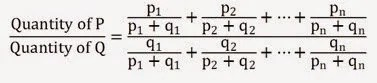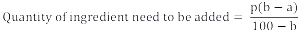# How To Solve Alligation Or Mixture Problems – Tips and Tricks

Alligation or Mixture problems are one of the time consuming problems. If you don’t know the exact method of solving these problem, you could end up getting wrong answers in the competitive examination. But the best part of quantitative aptitude is that once you know the shortcut and tips and tricks to solve any problem, its just a matter of second to solve any problems. Before continuing with the method to solve alligation or mixture problems, let’s learn some rule and definition :

Alligation : It is the rule that enables us to find the ration in which two or more ingredients at the given price must be mixed to produce a mixture of a desired price .

Mean Price (M) : The cost price of a unit quantity of the mixture is called the mean price.

Rule of Alligation : If two ingredients are mixed , then

It would be easier to understand by following diagram :

Now some some shortcut formulas to solve alligation or mixture problems fast :

Formula 1 :  If n different vessels of equal size are filled with the mixture of P and Q in the ratio p1 : q1,  p2 : q, ……, pn : qn and content of all these vessels are mixed in one large vessel, thenFormula 2 :  If n different vessels of sizes x1, x2, …, xn are filled with the mixture of P and Q in the ratio p1 : q, p2 : q2, ……, pn : qn and content of all these vessels are mixed in one large vessel, thenFormula 3 : Removal and Replacement Problems

If a vessel contains ‘x’ liters of liquid A and if ‘y’ liters be withdrawn and replaced by liquid B, then if ‘y’ liters of the mixture be withdrawn and replaced by liquid B, and the operation is repeated ‘n’ times in all, then :Formula 4 : If p gram or liters of ingredient solution has a% ingredient in it. Then to increase the ingredient content to b% in the solution , quantity of ingredient to be addedSo now we will solve problems based on the alligation or mixture ,

Example 1 : In what ratio must rice at Rs 9.30 per kg be mixed with rice at Rs 10.80 per kg so that the mixture be worth Rs 10 per kg ?

Solution 1 : This kind of question could directly be solved using rule of alligation , according to the given data :

Mean Price (M)  = Rs 10 per kg, Cost Price of Costlier (D) = Rs 10.80 per kg , Cost Price of Cheaper (C) = Rs 9.30 per kg

Let’s use our rule of alligation :

Quantity of Cheaper / Quantity of Dearer = (CP of Costlier – Mean Price ) / (Mean Price – CP of Cheaper )

Therefore, Required ratio = (10.80 – 10) /  (10 – 9.30) = 0.80 / 0.70 => 8 : 7

Example 2In what ratio must a person mix three kinds of wheat costing him Rs 1.20, Rs 1.44 and Rs 1.74 per Kg so that the mixture may be worth Rs 1.41 per Kg?

Solution 2 : This is also solved using rule of alligation but it involves concept of ratio and proportion too.

Since there are more than two kinds of wheat present here , we need to find the ratio between pairs separately. Assume CP of 1st kind (Rs 1.20 per kg ) as I , CP of 2nd kind (Rs 1.44 per kg ) as II per kg and CP of 3rd kind (Rs 1.74 per kg ) as III.

Hence using rule of alligation,

I : II = (1.44 – 1.41) / (1.41 – 1.20) = 0.03 / 0.21 => 1 : 7

Similarly, I : III = (1.74 – 1.41) / (1.41 – 1.20) = 0.33 / 0.21 => 11 : 7

By using concept of Ratio and Proportion, II : III = (I / III ) / (I / II) = (11 / 7) / (1 / 7) => 11 : 1 = (11 x( 7/11)) : (1 x( 7/11)) = 7 : (7/11)

Therefore, Required Ratio , I : II : II = (1 x 11) : (7 x 11) : 7 => 11 : 77 : 7

Example 3A painter mixes blue paint with white paint so that the mixture contains 10% blue paint. In a mixture of 40 liters paint how many liters of blue paint should be added so that the mixture contains 20% of blue paint ?

Solution 3 : This problem is also solved using rule of alligation but there is also a direct formula to get the answer. The above problem suits to the direct formula number 4 given above.

Here p = 40 liters, a = 10, b = 20

Quantity of liters of blue paint to be added = p(b-a) / (100 -b) = 40 * (20 – 10) / (100 – 20) => 5 liters

Example 4A milk vendor has 2 cans of milk .The first contains 25% water and the rest milk. The second contains 50% water. How much milk should he mix from each of the container so as to get 12 liters of milk such that the ratio of water to milk is 3:5?

Solution 4 :  This problem is solved by getting ratio of milk in 1st can to  the milk in 2nd can

Milk in 1st can = 75% = 3/4 (Dearer amount, D)

Milk in 2nd can  = 50% = 1/2 (Cheaper amount, C)

Milk in the mixture = 5 / (3+5) = 5/8 ( Mean, M)

Therefore, Quantity of Cheaper / Quantity of Dearer = (D – M ) / (M – C) = ((3/4) – (5/8)) / ((5/8)-(1/2)) = 1/8 / 1/8 => 1:1

Let x amount of milk to be added from the 1st can, thus x amount of milk would be added from 2nd can because both are in the same ratio

Thus x + x = 12 liters => x = 6 liters. There 6 liters of milk to be added from both the containers to get 12 liters of milk in the resultant mixture.

Example 5 : A can contains a mixture of two liquids A and B in the ratio 7:5 . When 9 liters of mixture are drawn off and the can is filled with B, the ratio of A and B becomes 7:9. How many liters of liquid A was contained by the can initially ?

Solution 5 :  Suppose the can initially contains 7x and 5x liters of mixtures A and B respectively, Therefore after withdrawn of 9 liters of mixture

Quantity of A in mixture left = ((7x) – ((7/12) * 9)) => 7x – (21 / 4) liters

Quantity of B in mixture left = ((5x)-((5/12) * 9)) => 5x – (15 / 4) liters

Its given ratio of A and B in mixture is 7 : 9, Hence

(7x – (21/4)) / ((5x) – (15/4)) = 7 / 9 => x = 3

Thus initially can contains 7x liters of A = 21 liters

Example 6 : The milk and water in two vessels A and B are in the ratio 4 : 3 and 2 : 3 respectively. In what ratio, the liquids in both the vessels be mixed to obtain a new mixture in vessel C containing half milk and half water ?

Solution 6 : Assume CP of milk = Rs 1 per liter

Therefore Milk in 1 liter of mixture of A = 4 / 7 liter , in 1 liter of mixture of B = 2 / 5 liter

Milk in 1 liter of mixture of C = 1/2

Therefore , CP of 1 liter of mixture in A = Rs 4 / 7 (Costlier CP D),  CP of 1 liter of mixture in B = Rs 2 / 5 (Cheaper CP C) ,

CP of 1 liter of mixture in C = Rs 1/2  ( Mean Price M)

Now using rule of Alligation, Required ratio

Quantity of mixture in A / Quantity of mixture in B =  (D-M) / (M-C) = (4/7 – 1/2) / (1/2 – 2/5) => 7 : 5

Example 7 : A and B are two alloys of gold and copper prepared by mixing metals in the ratio 7 : 2 and 7 : 11 respectively. If equal quantities of the alloys are melted to form a third alloy C, the ratio of gold and copper in C will be ?

Solution 7 : Use the Formula Number 1 given above in the post ;

Therefore, quantity of Gold in C = (7/9 + 7/18) units = 7/6 units

quantity of Copper in C = (2/9 + 11/18) = 5/6 units

Therefore, Gold : Copper  in resultant alloy C = 7/6 : 5/6 => 7 : 5

#### 1 COMMENT

This site uses Akismet to reduce spam. Learn how your comment data is processed.